Home » Math » 50 Hands- On And Fun Multiplication and Division Activities

# 50 Hands- On And Fun Multiplication and Division Activities

Learning math while playing games and working puzzles is so much fun! And these 50 multiplication and division activities will bring fun to math time.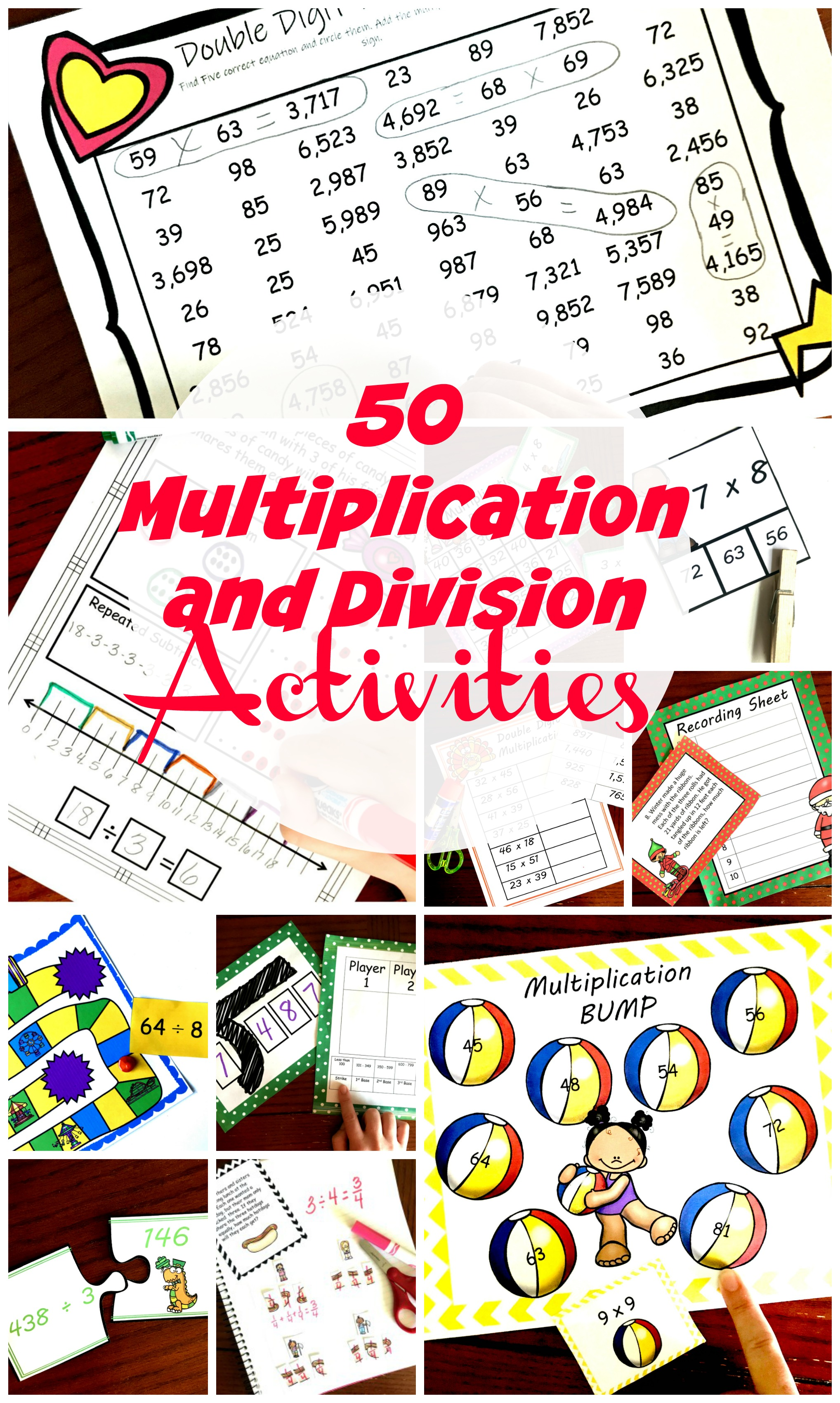Just as adding and subtracting are important skills in early elementary, conquering multiplication and division are just as important as children get older.

But very few children want to sit and study flashcards. So this page has multiple resources that can help make learning multiplication and dividing fun.

They can learn to skip count, play games to work on memorizing their math facts, or just practice their long multiplication and division skills through puzzles and other fun worksheets.

Have fun exploring these 50 multiplication and division activities.

Are you seeing a lot of activities that you want?

This bundle has them all in one easy download.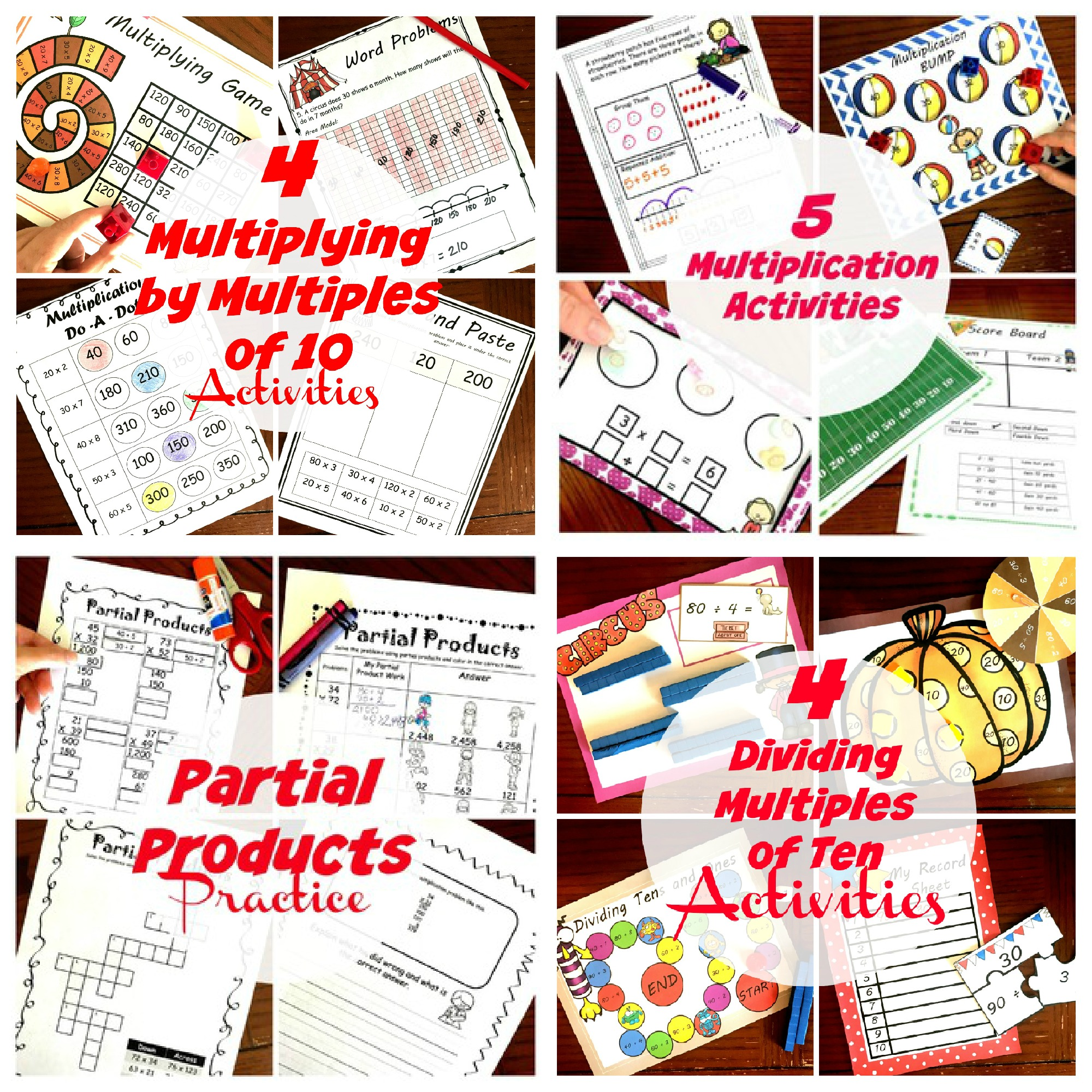## Multiplication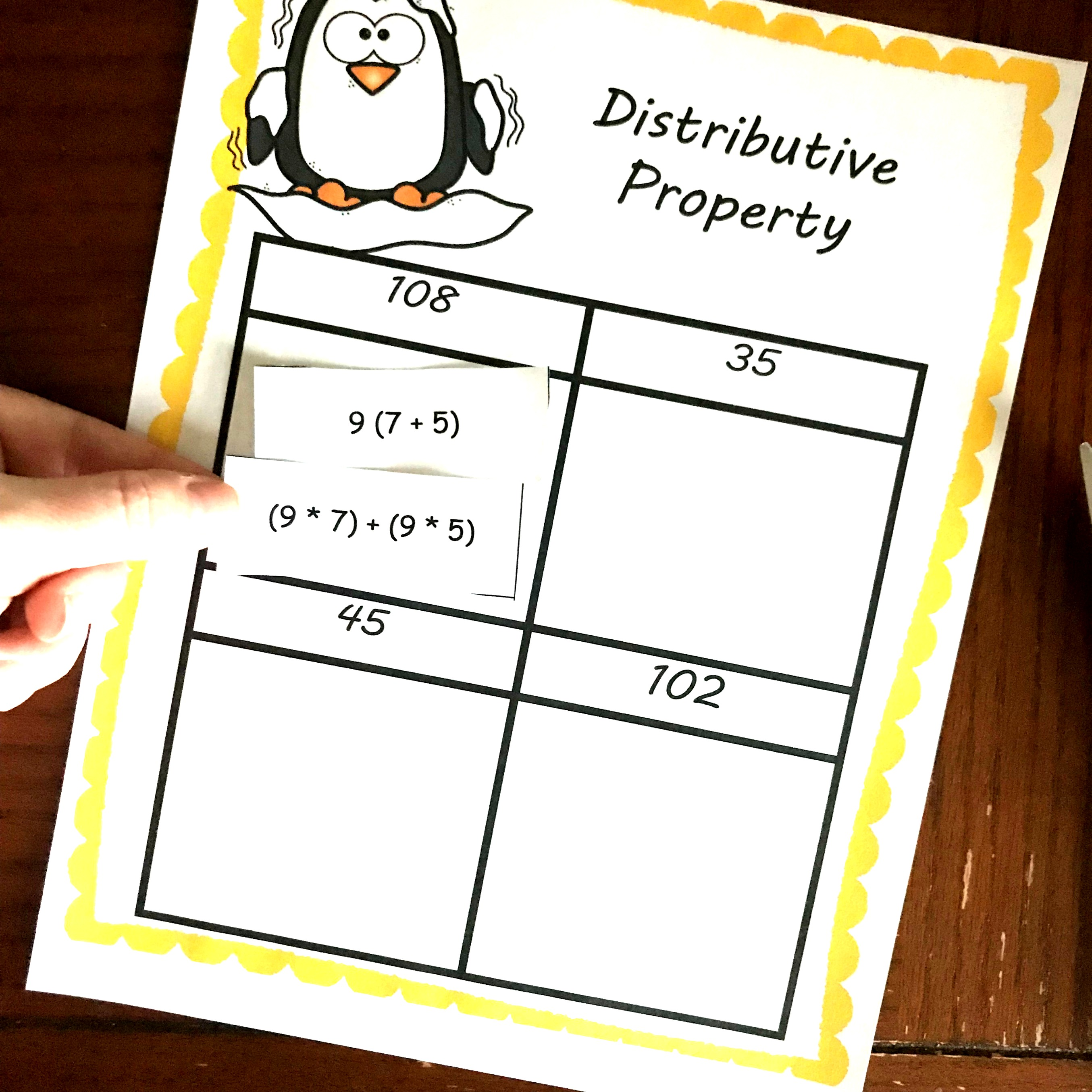THREE, FREE Distributive Property Worksheets with a Winter Theme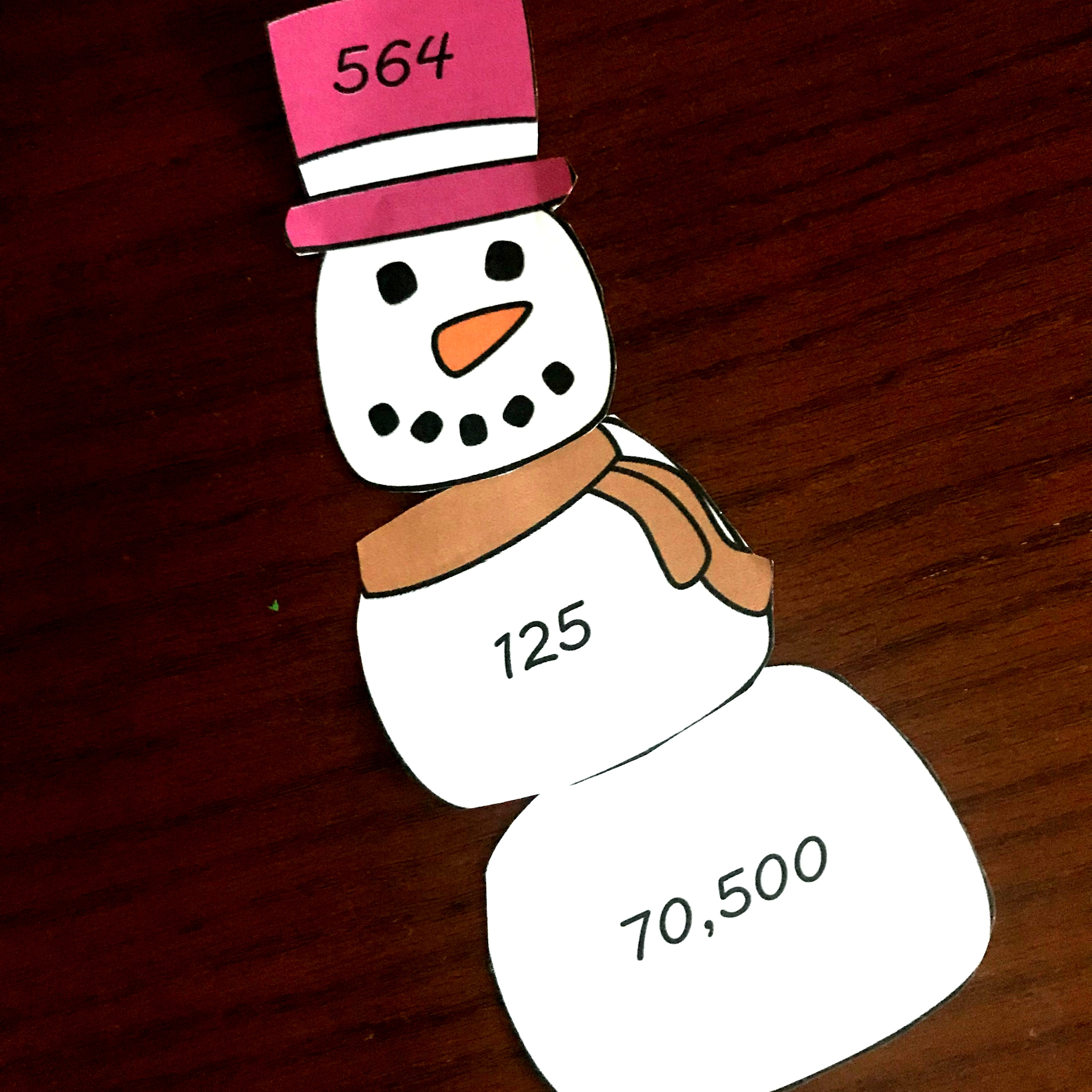FREE Winter Activity For 3 Digit by 3 Digit MultiplicationFREE Challenging Christmas Multiplication and Division Word ProblemsFREE Two Digit Multiplication Worksheets with a Fun TwistFREE Cut and Paste Double Digit Multiplication WorksheetsA FREE Delightful Game For Multiplying by Multiples of 10FREE Interactive Multiplication Word Problems WorksheetsFREE Low-Prep Times Table Game For Kids To Memorizing Facts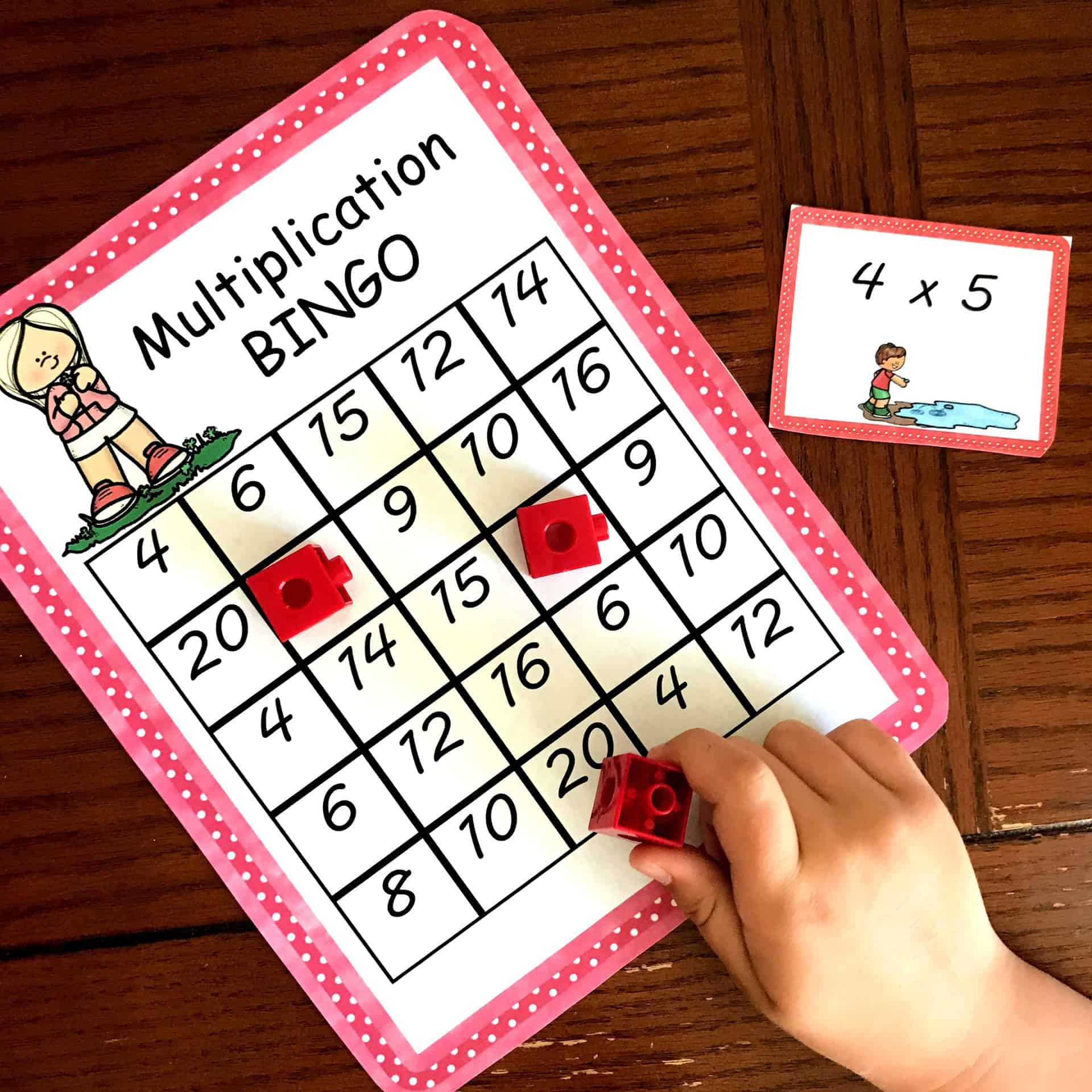Fun Multiplication Games to Help Children Learn Their Multiplication Facts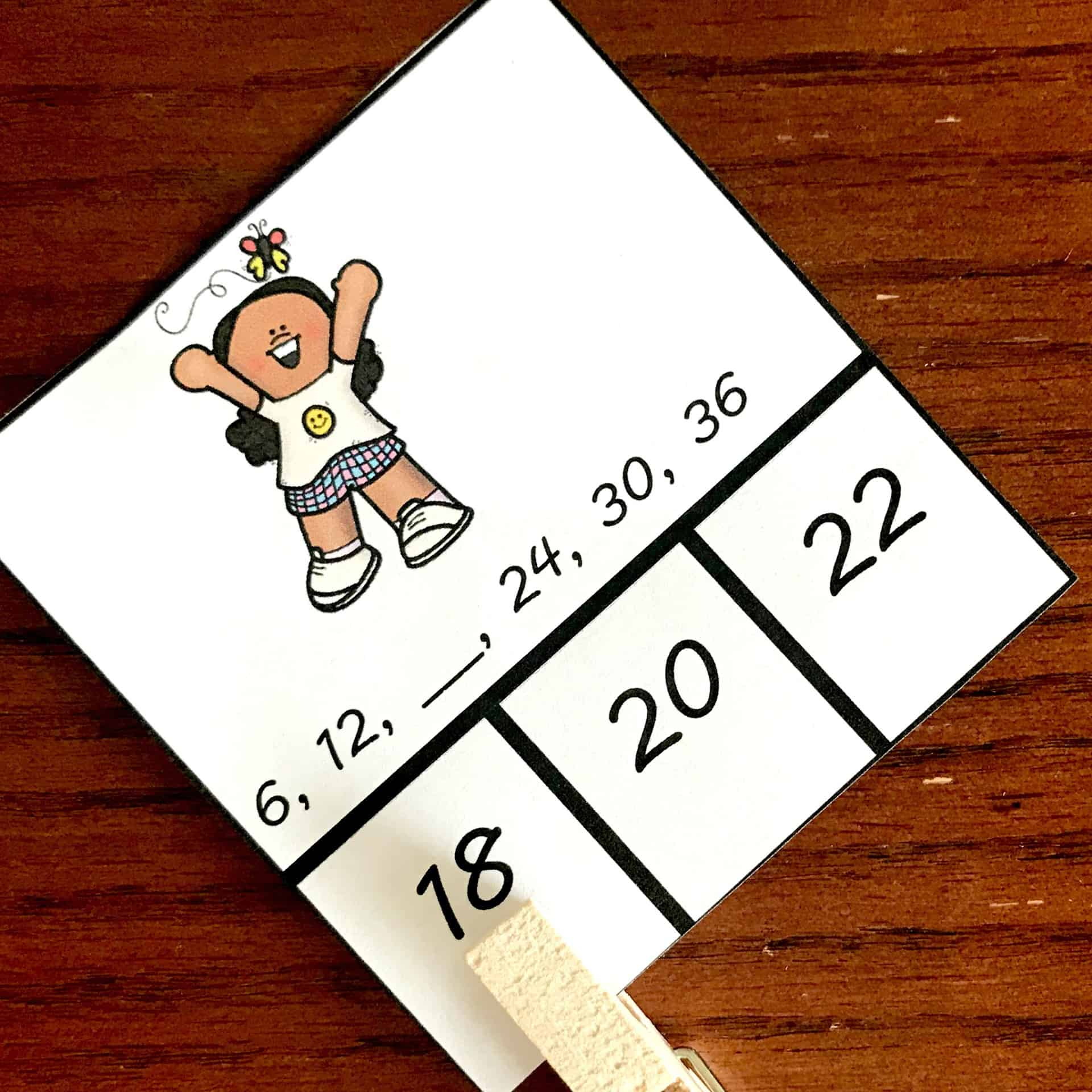60 Skip Counting Clip Cards for The Numbers Two Through Fifteen15 Skip Counting Mazes for Numbers Two Through Fifteen15 Skip Counting Puzzles To Build Schemas For Multiplication Facts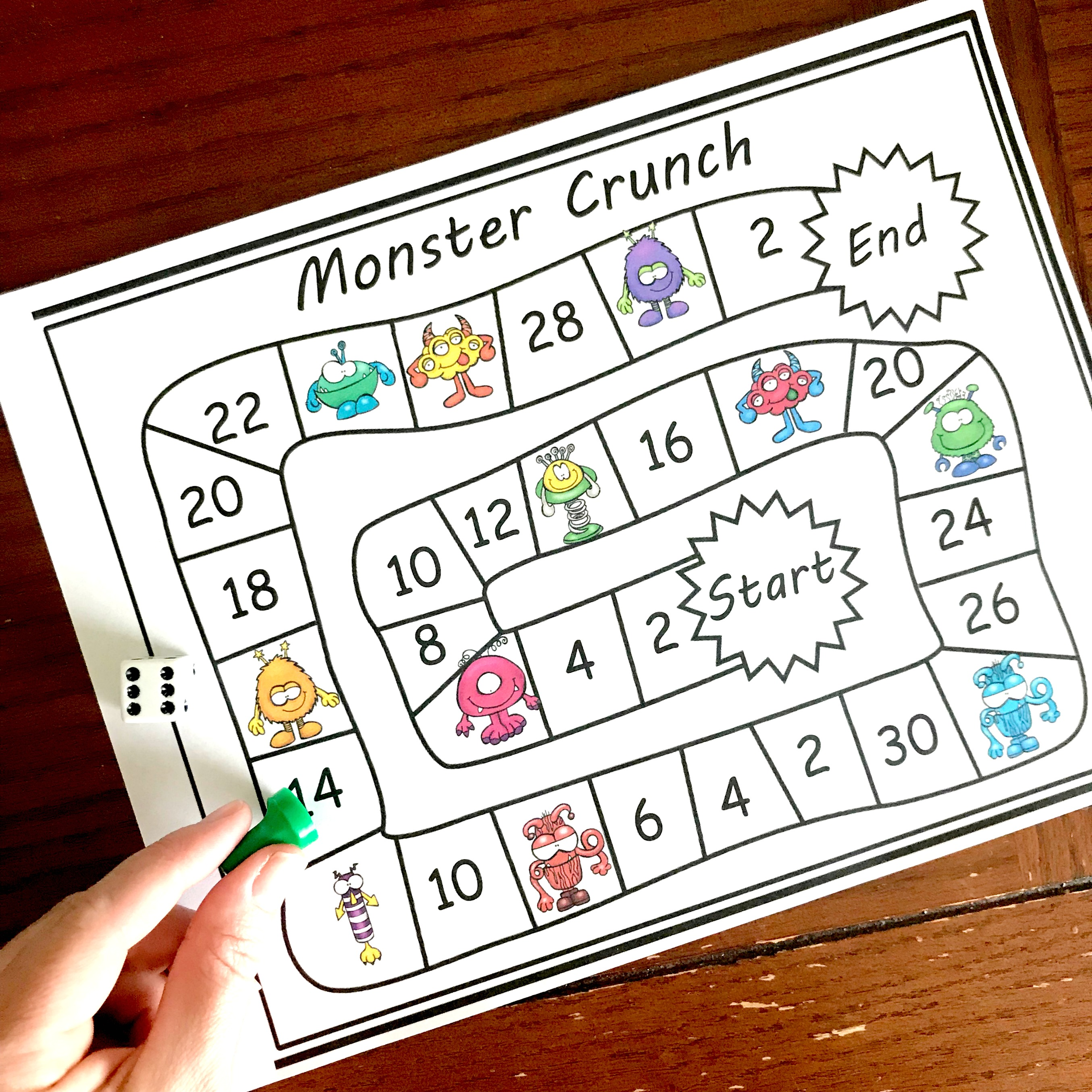Grab these Skip Counting Games for the Classroom – Practice 2’s to 15’s!10 Multiplying Fractions Word Problems For Some Real Life Examples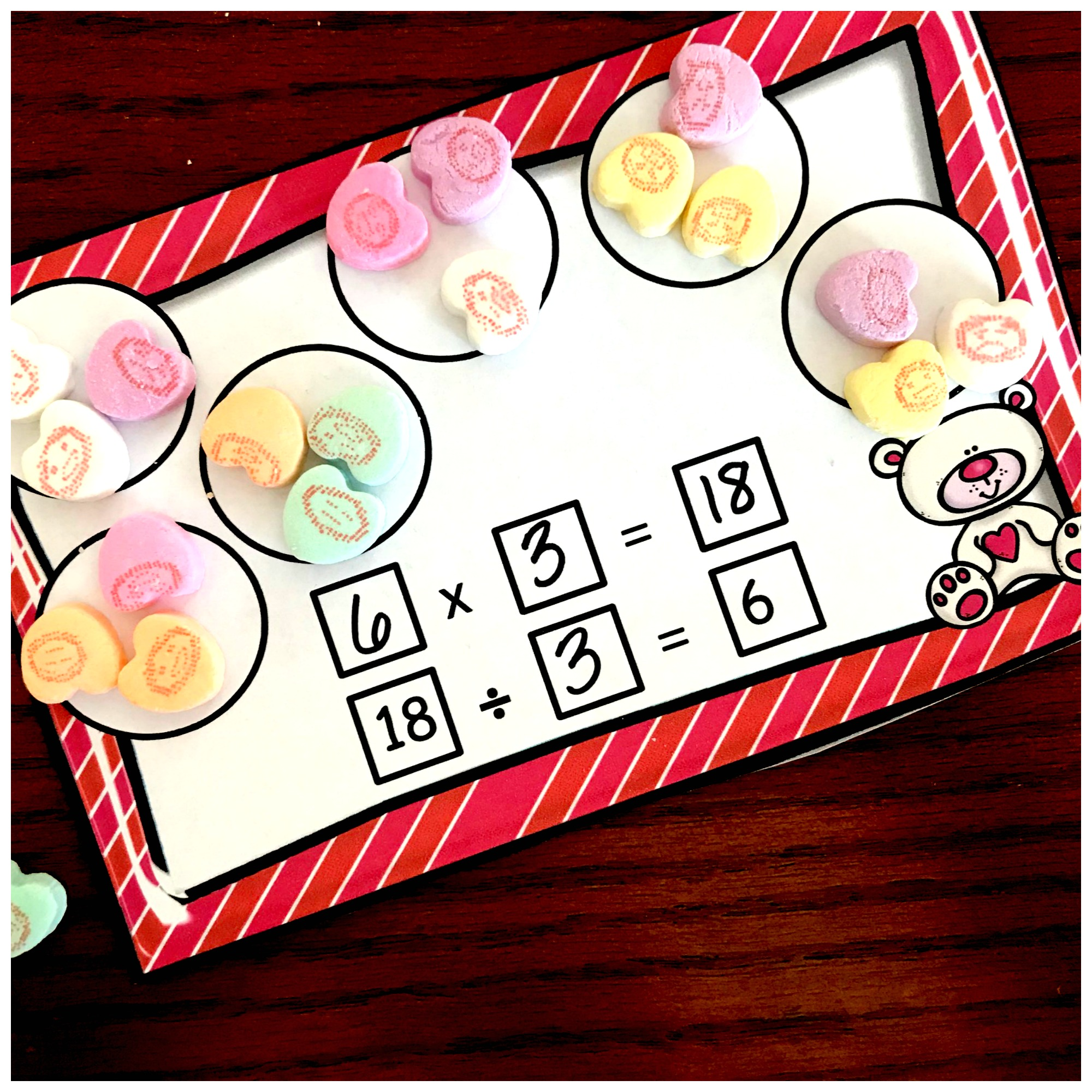Here’s FREE Missing Factor Worksheets or Task Cards For Extra PracticeFree Printable Fraction Game For Multiplying Fractions3 Cut and Paste Worksheets For Multiplying Fractions Practice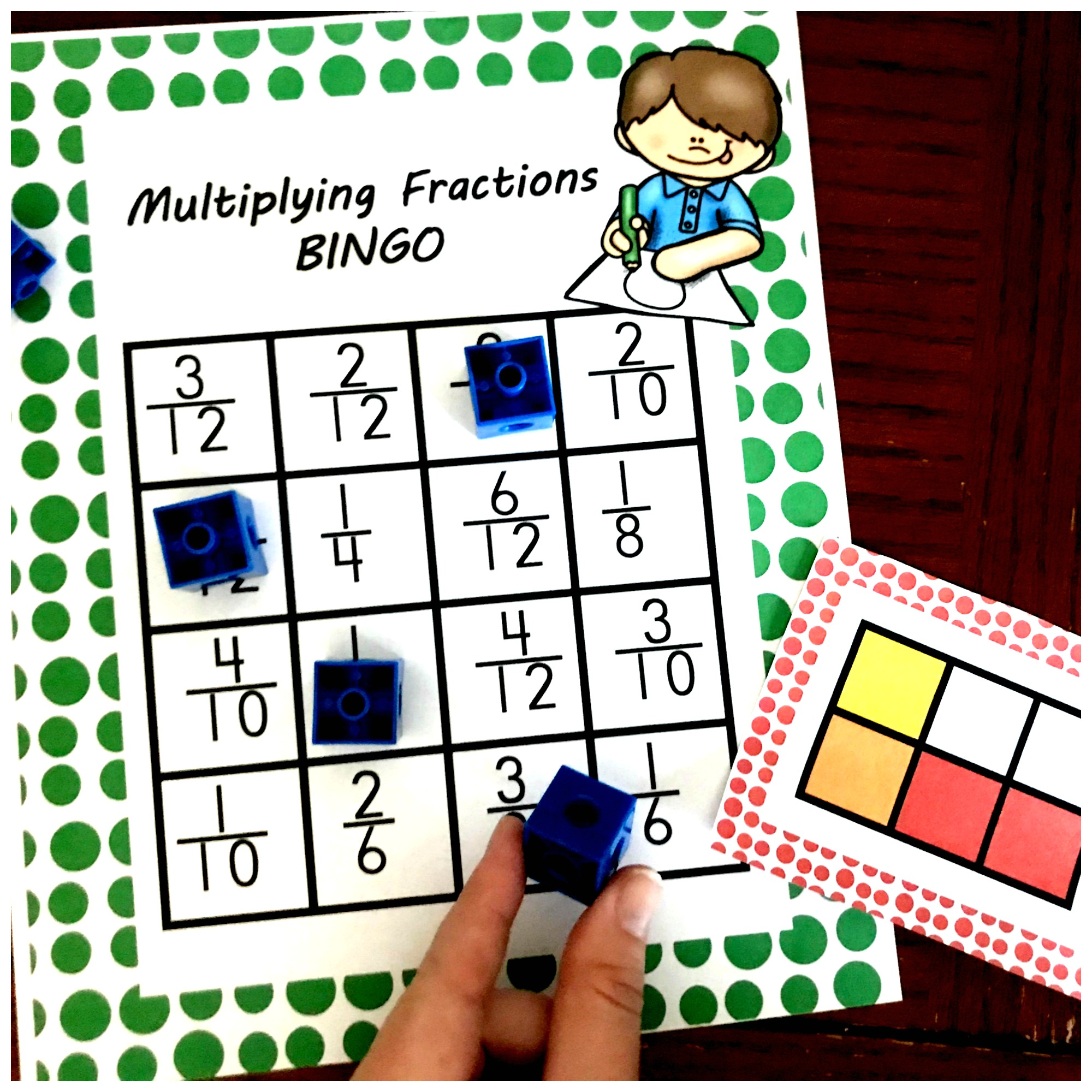Here’s a Multiplying Fractions Game That’s Perfect for Extra PracticeOne Free, Hands – On Multiplying Fractions ActivityHow to Teach Lattice Multiplication: Includes a Free Step by Step PrintableHow to Teach Large Digit Multiplication Using Arrays and Base Ten BlocksHow To Teach Multiplication Using Area Model (Free Printable)Four Ways To Teach Multiplication with Free A Printable – Part 1 Partial Products20 Word Problems for Multiplying Fractions By Whole NumbersMultiplying Fractions on a Number Line GameFREE Multiplying Fraction Activity To Teach Whole Numbers by Fractions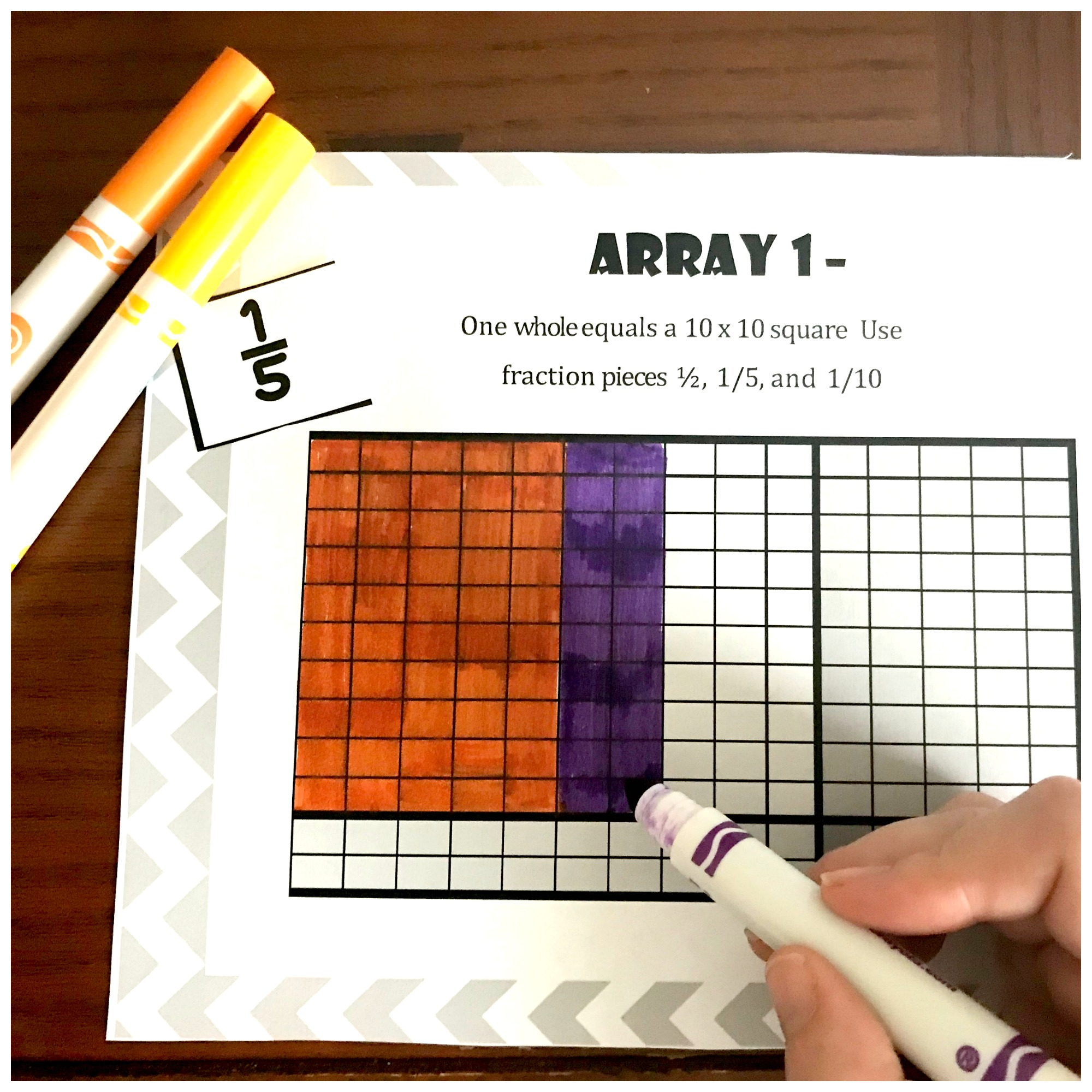Here’s a Free Game To Teach Multiplying Fractions With ArraysOne Word Problem to Further Explore Area and Perimeter

Books About Multiplication from the Measured Mom

Double Digit Multiplication Practice from Math Geek Mama

Multiplication Fact Clip Cards from Math Geek Mama

Editable Math Facts Game from Playdough To Plato

Multiplication Pumpkin Puzzles from 123homeschool4me

Word Problems with Multiplication from The Measured Mom

More Multiplication Word Problems from Playdough to Plato

No-Prep Multiplication Game From This Reading Mama

## Division50 Hands- On And Fun Multiplication and Division Activities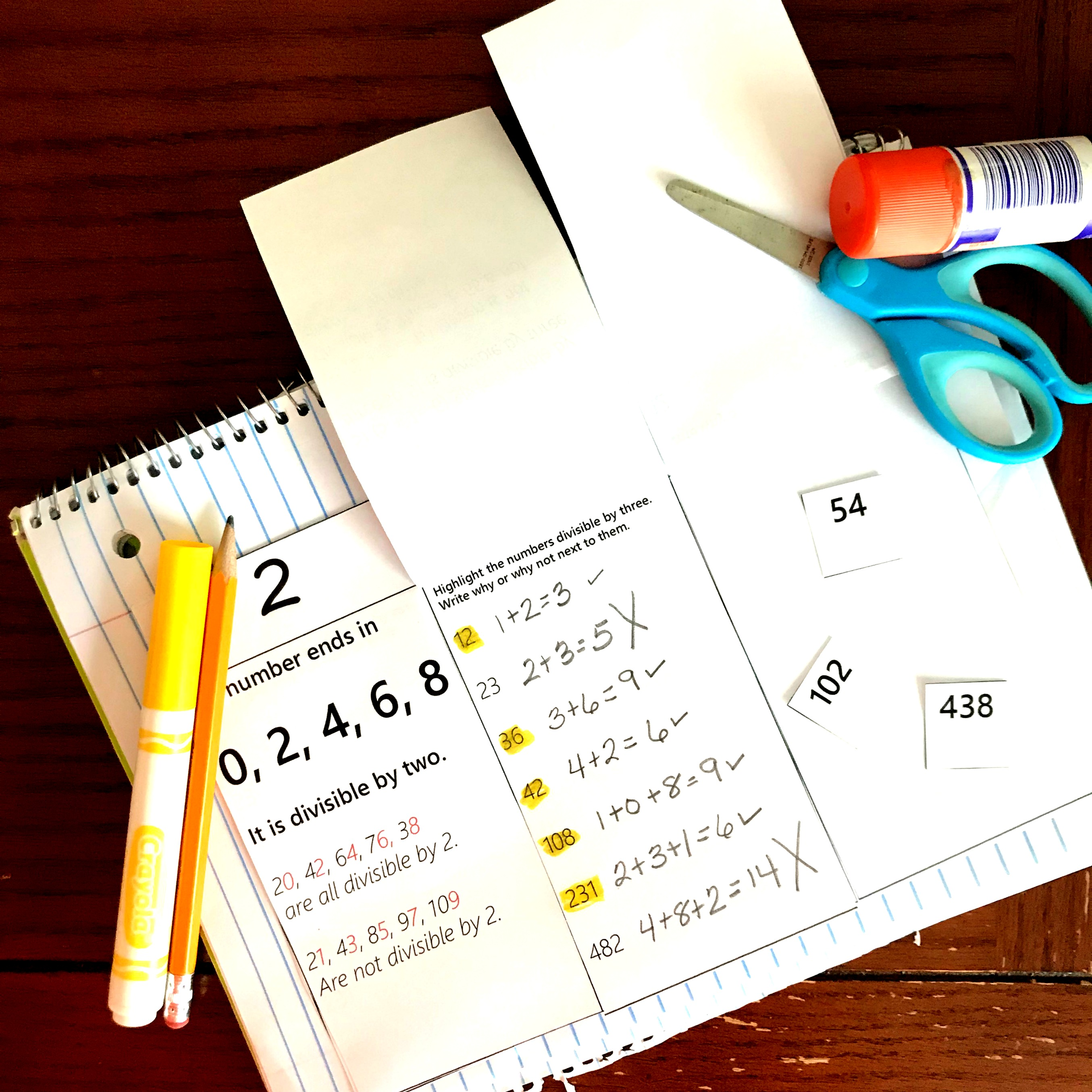FREE Hands- On Divisibility Rules Interactive Notebook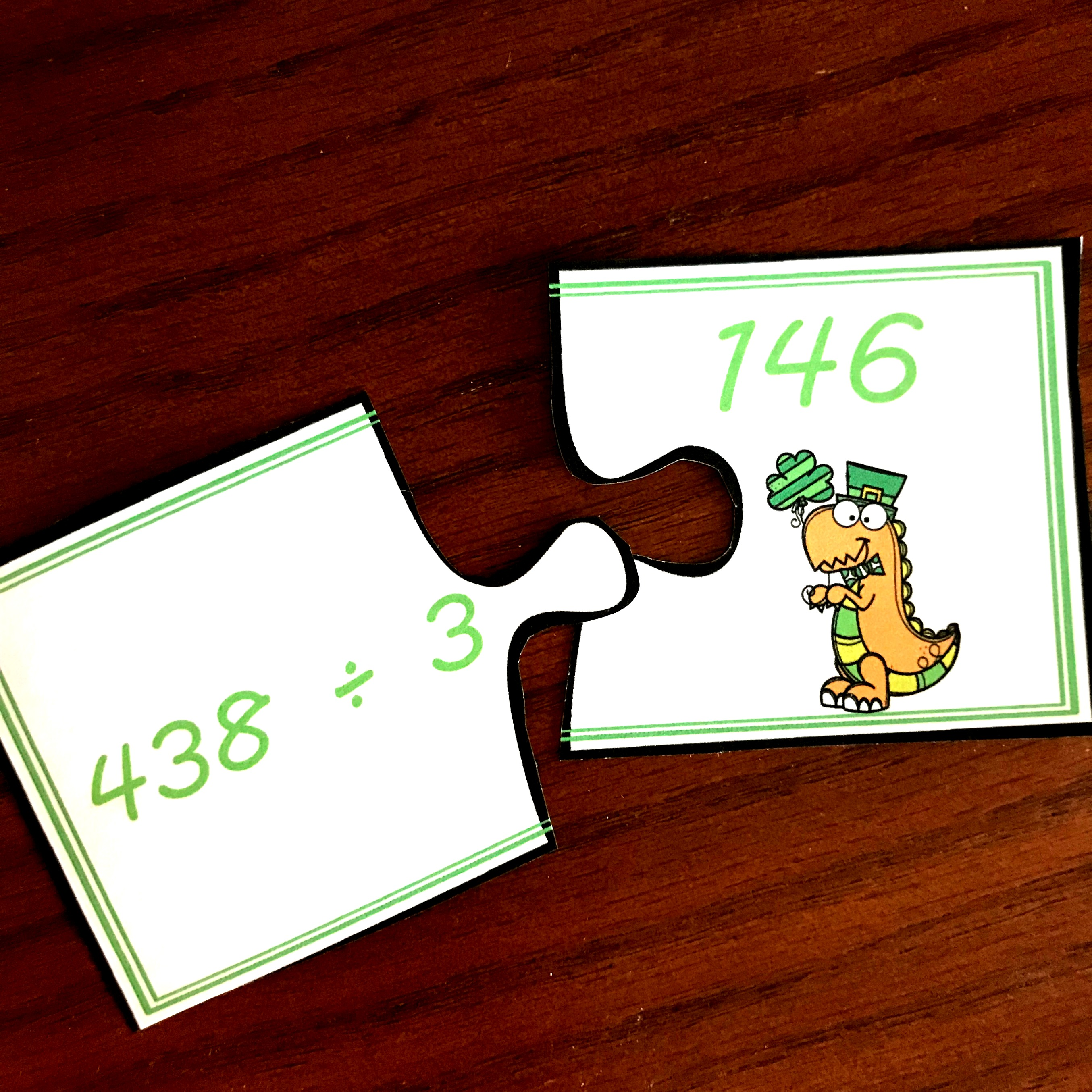FREE Puzzles For Long Division PracticeFREE Divide using Partial Quotients Cut and Paste ActivityFREE Valentine’s Day Dividing Whole Numbers by Decimals Worksheet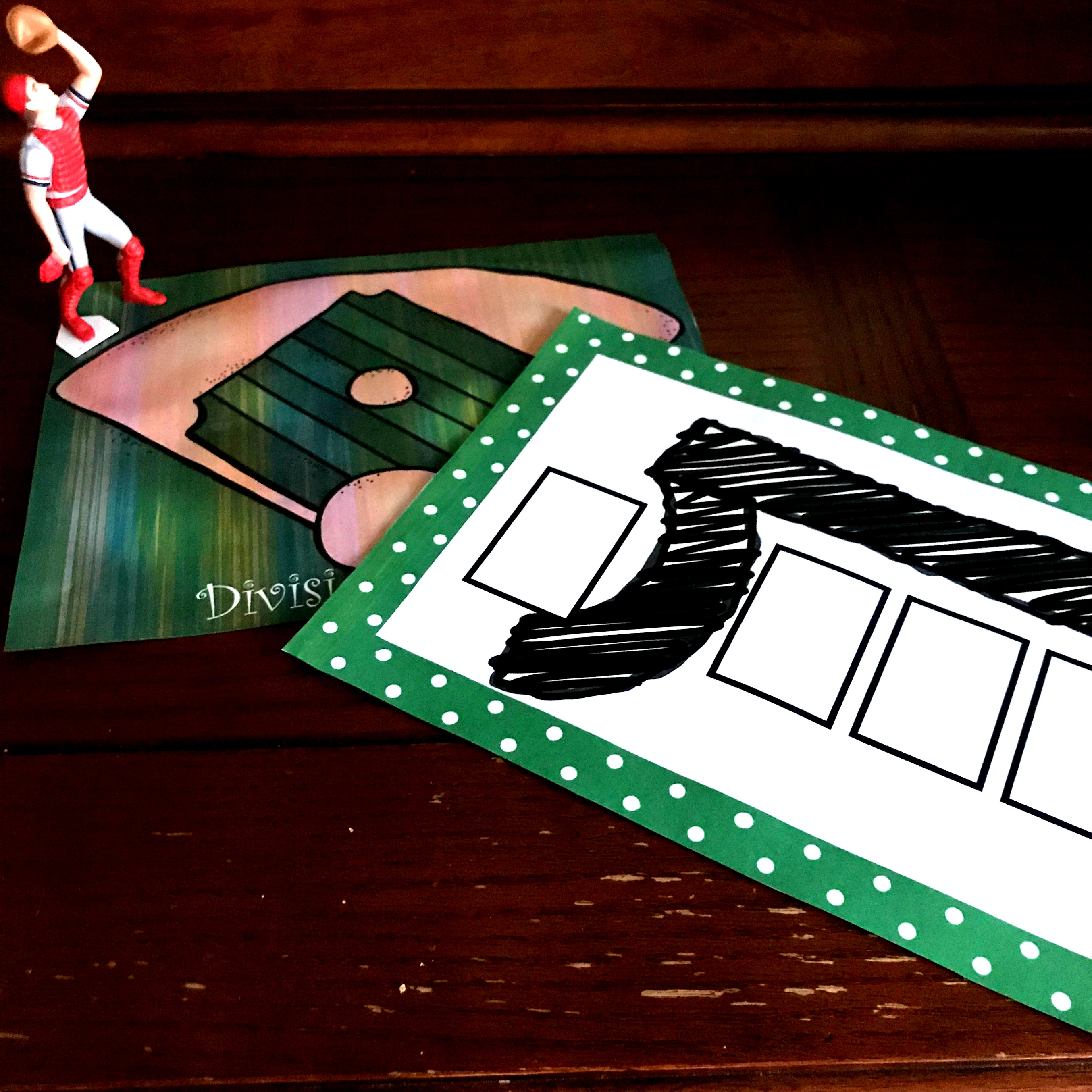FREE No-Prep Long Division Game to Practice this Challenging SkillFREE Challenging Christmas Multiplication and Division Word ProblemsFREE No-Prep Christmas Game For Dividing Multiples of 10 by a Single Digit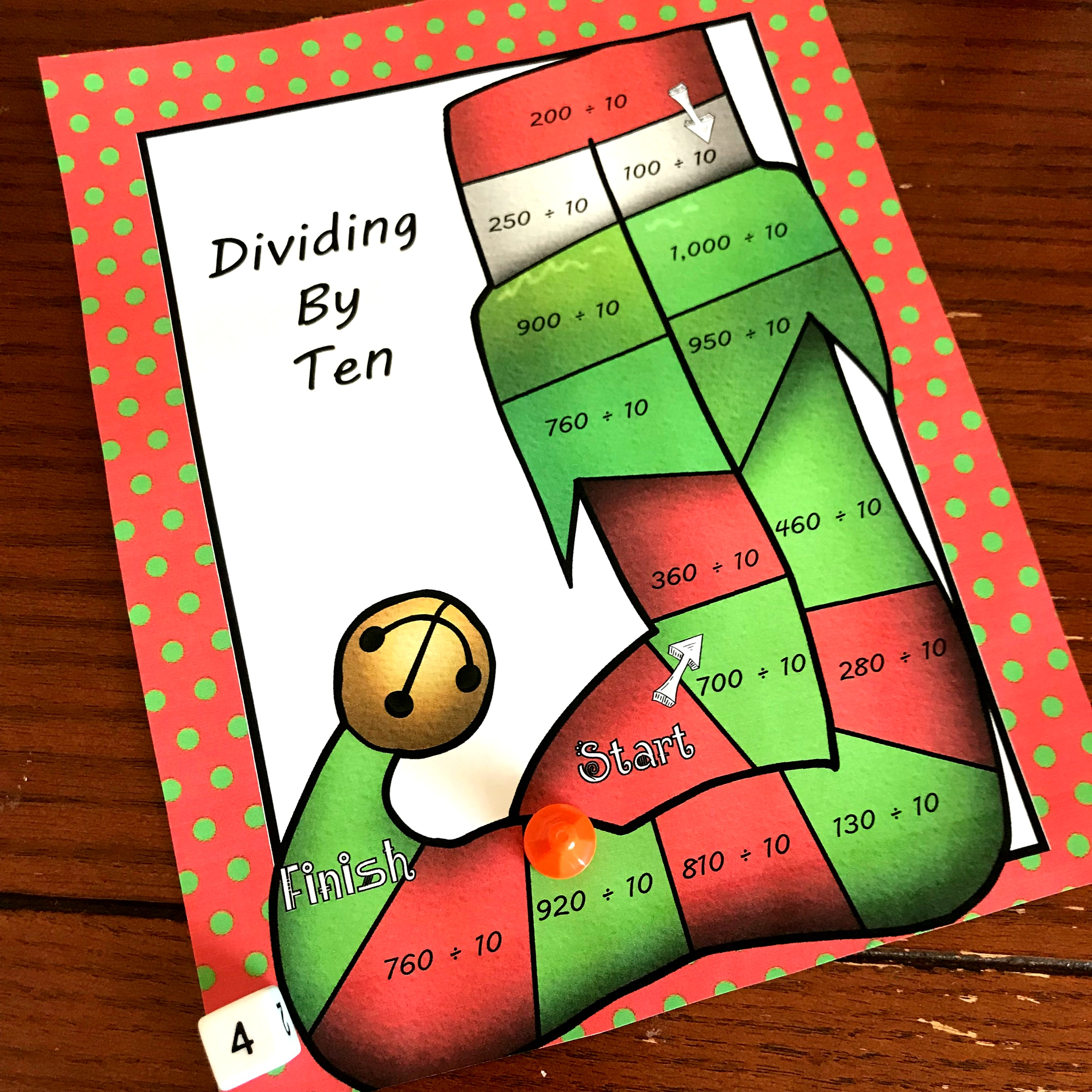FREE No-Prep Dividing By 10 Game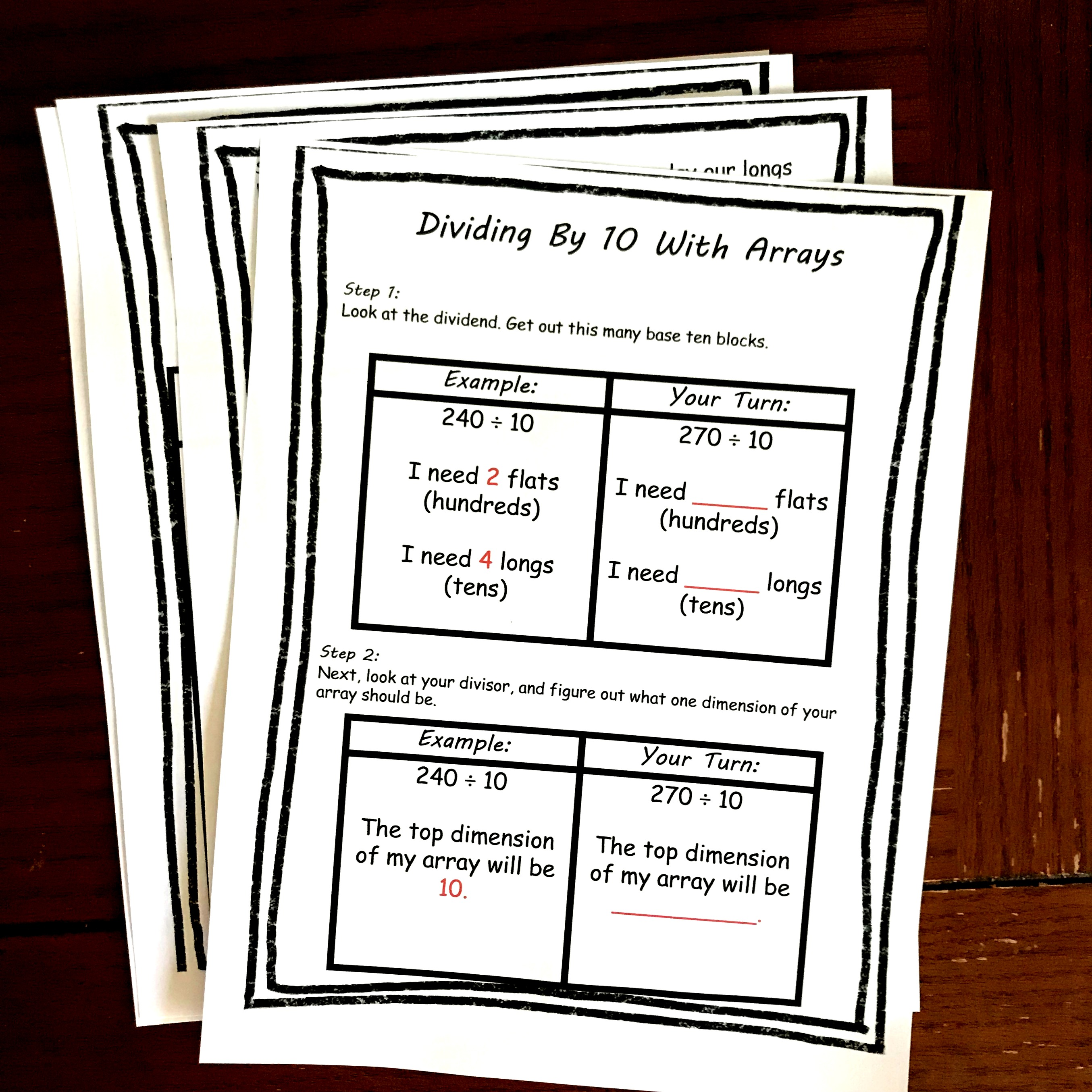FREE Step by Step Directions For Dividing by 10 Using ArraysFREE Fun Division Game For Practicing Basic Division FactsFREE Low-Prep, Spin and Cover Dividing Multiples of Ten GameFREE Yummy Word Problems For Dividing Fractions by FractionsHere’s FREE Missing Factor Worksheets or Task Cards For Extra Practice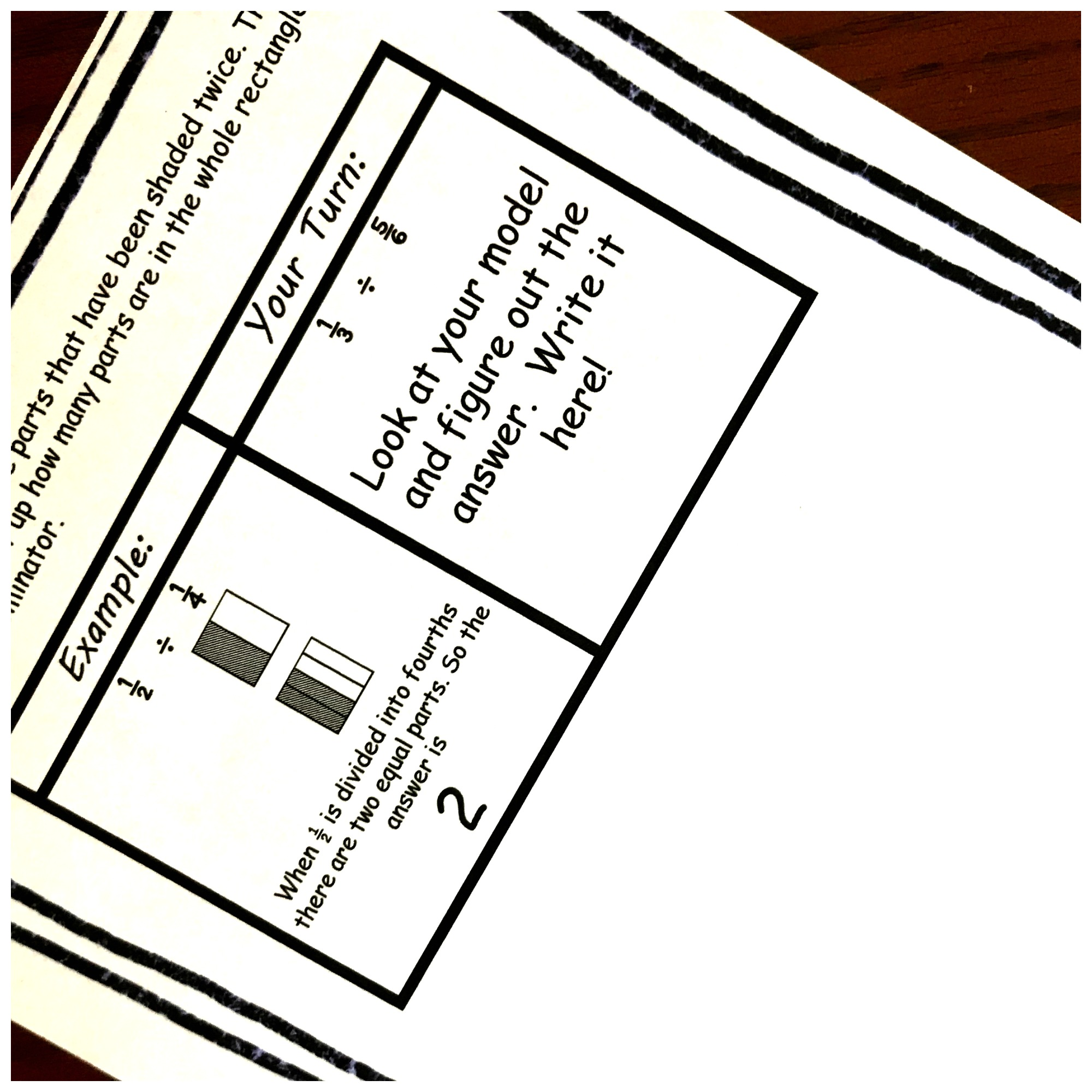FREE Interactive Notebook To Provide Dividing Fractions Examples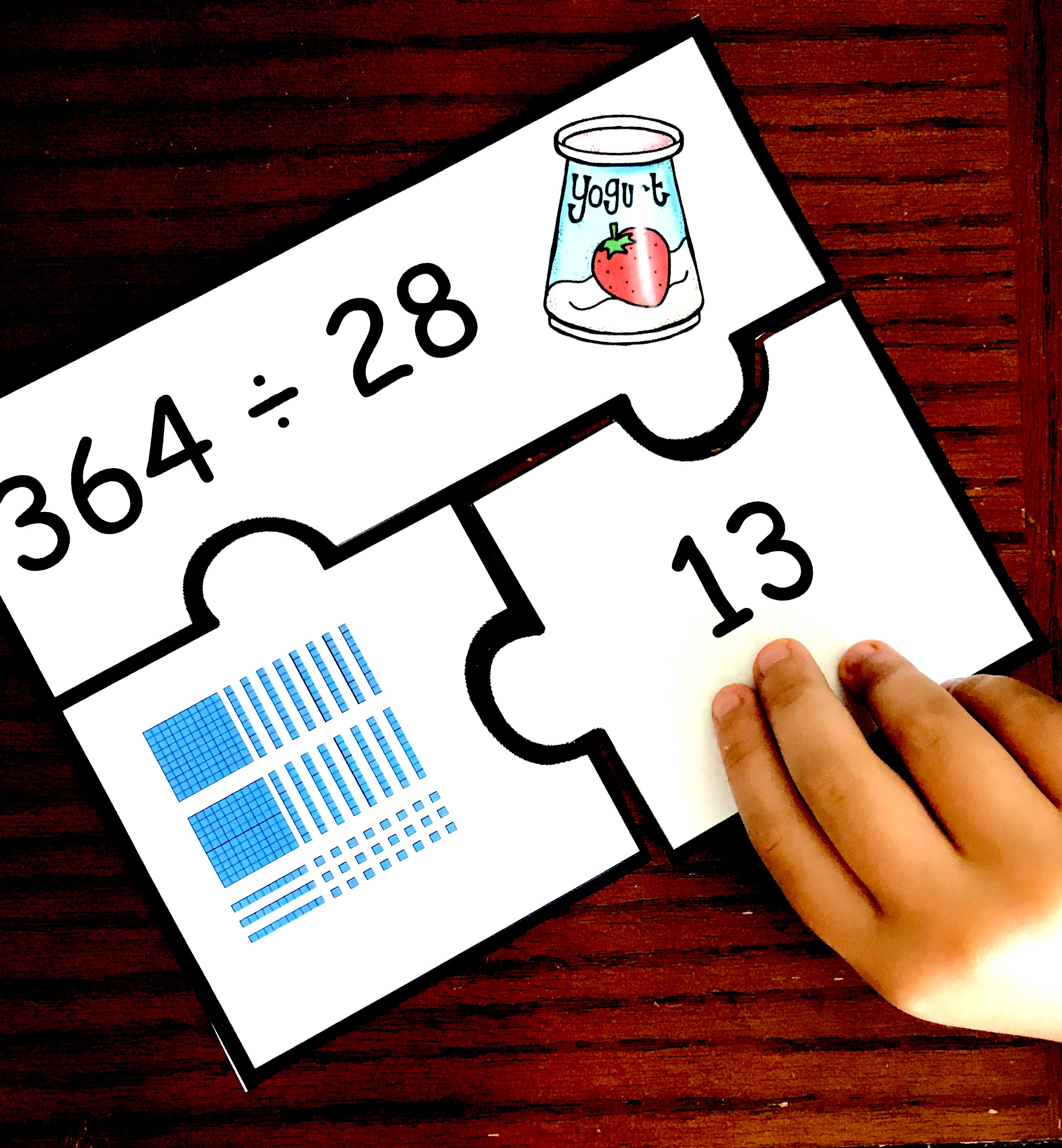How to Practice Long Division in a Fun, Engaging Way (Free Division Puzzles)Grab Your Free Two Step Word Problems with a Patriotic ThemeFREE Division Word Problems with Five Strategies To SolveHow to teach divison using arrays (FREE interactive notebook)How to Teach Division of Whole Numbers Leading to Answers with Fractions

Equal Sharing Task Cards from the Measured Mom

Division Word Problems within 20 from Playdough to Plato

Single Digit Divison Game by Math Geek Mama

Multiplication and Division Fact Family Pack from This Reading Mama

Long Division Practice offered by Math Geek Mama

Deck Of Cards Division by 123Homeschool4me

## Multiplying and Dividing Fractions10 Multiplying Fractions Word Problems For Some Real Life ExamplesFree Printable Fraction Game For Multiplying Fractions3 Cut and Paste Worksheets For Multiplying Fractions PracticeHere’s a Multiplying Fractions Game That’s Perfect for Extra PracticeOne Free, Hands – On Multiplying Fractions Activity20 Word Problems for Multiplying Fractions By Whole NumbersMultiplying Fractions on a Number Line GameFREE Multiplying Fraction Activity To Teach Whole Numbers by FractionsHere’s a Free Game To Teach Multiplying Fractions With ArraysOne Word Problem to Further Explore Area and Perimeter

I hope you enjoy these activities, and your kiddos have fun mastering multiplication and division.

### 2 thoughts on “50 Hands- On And Fun Multiplication and Division Activities”

1.Schuele Batenhorst says:

How can I access the bundled download? So many of these activities are amazing and I’d love to be able to grab them all at once!

1.Rachel C says:

I’m sorry about that. I have the pictured linked now. You can get the multiplication and division bundle here. https://www.teacherspayteachers.com/Product/Multiplication-and-Division-Bundle-4163282